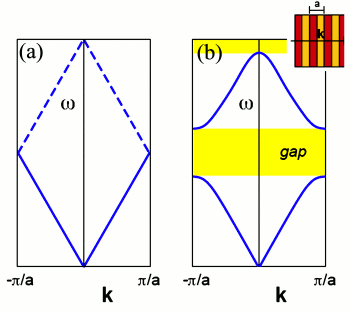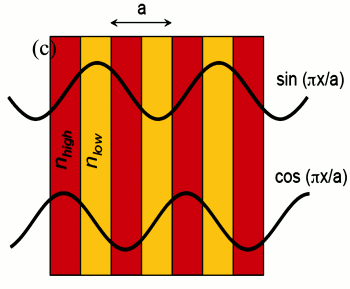Manipulating Light with Photonic Crystal

Bing Dai May 21, 2008

(Submitted as coursework for Applied Physics 273, Stanford University, Spring 2007)Fig. 1: (a) Band diagram of a uniform one-dimensional medium, where the dashed lines show the folding effect of applying the Bloch theorem with an artificial periodicity a. (b) Schematic effect on the bands of a physical periodic dielectric variation (inset), where a gap has been opened by splitting the degeneracy at the f = ±π/a, the 1D Brillouin zone boundaries.

Photonic crystals are periodically structured electromagnetic media. The periodicity, whose lengthscale is proportional to the wavelength of light of interest, is the electromagnetic analogue of a crystalline atomic lattice, where the latter acts on electron wavefunction to produce the familiar band gaps, semiconductors, etc., of solid state physics. Like we can control the behavior of electrons in semiconductor, we can also control the properties of electromagnetic waves by modeling the structure of photonic crystals. In this report, we will study the physical origin of photonic band gap and discuss how we can manipulate the propagation of light.

Maxwell's Equations in Periodic Media

To start with, let's study the propagation of light in a photonic crystal by the Maxwell equations. If we restrict ourselves to linear, lossless materials and assume that the Maxwell equations impose on a field pattern that happens to vary sinusoidally (i.e. in harmonic modes) with time, we can separate out the time dependence of electric and magnetic fields, i.e., H(r,t) = H(r)e-iωt. Then the Maxwell equations for the steady state are ,

∇ × H(r) + (ε(r0E(r)) = 0
∇ × E(r) = 0H(r))

Express the equation in magnetic field only:

∇ × (1/ε(r)) × H(r) = (ω/c)2H(r)

This is the master equation. Let operator Θ = ∇ × (1/ε(r)) ×, then the master equation becomes

ΘH(r) = (ω/c)2

Then it becomes an eigenvalue problem, with (ω/c)2 being the eigenvalues and H(r) the eigenvectors (modes). We can further prove that Θ is a Hermitian. So solutions H(r) are orthogonal and ω2 are real and positive, and they can be obtained by a variational principle.

Now we use the variational theorem to study the EM distribution of modes in the dielectric media. The lowest-frequency mode is the field pattern that minimizes the EM energy functional:

Ef(H) = ½(HH)/(H,H) = 1/(2(H,H)) ∫dr(1/ε)|(ω/c)D|2Fig. 2: Schematic origin of the band gap in one dimension. The degenerate k = ±π/a plane waves of a uniform medium are split into cos(πx/a) and sin(πx/a) standing waves by a dielectric periodicity, forming the lower and upper edges of the band gap, respectively; the former has electric-field peaks in the high dielectric (nhigh) and so will lie at a lower frequency than the latter which peaks in the low dielectric region.

Add a small variation δH(r) to H(r), it can be proved that Ef(H + δH(r)) = Ef(H) when H is an eigenvector of Θ, i.e., Ef(H) is stationary with H. Therefore, solutions (modes) to the master equation are stationary points of the functional. And we can see from the last equation that Ef is minimized when the field is concentrated in the region of dielectric constant. Therefore, lower order modes tend to concentrate its displacement field in regions of high dielectric constant. This conclusion provides us a simple way to qualitatively understand the features of modes, i.e., band diagram, in the dielectric media.

Origin of Photonic Band Gaps

A photonic crystal has periodical dielectric constant ε(r) = ε(r + Ri), Ri is the primitive lattice vectors. Based on the Bloch theorem, the master equation will have solutions in form of Hn(r) = eikr, where uk(r + Ri) = uk(r), with eigenvalues ωn(k) satisfying ωn(k + Gj), where Gj is the primitive reciprocal lattice defined by RiGj = 2πδi,j. Since ωn(k) are periodic, we can plot ω vs k in the first Brillouin zone to get the band diagram of photonic crystals.

Like the band gap of semiconductor, photonic crystals also have band gaps in which there are no propagating solutions (real k) of the master equation. We will discuss the origin of photonic band gaps in the rest of the section. For simplicity, we examine the one-dimensional system.

Consider a 1D system with uniform dielectric constant ε. Then the eigenvectors are ω(k) = ck, as depicted in Fig. 1a and the E-fields are E(x) ∼ e±kx. We set an artificial periodicity a to fold the bands to the Brillouin zone (as shown by the dashed lines in Fig. 1a). So now the k = -π/a mode lies at an equivalent wavevector to the k = π/a mode, and at the same frequency. So there is a degeneracy at the zone boundary, k = π/a. We rewrite the E-fields at the boundary to be e(x) = cos(πx/a) and o(x) = sin(πx/a) (shown in Fig.1(c)), a linear combination of E(x) ∼ e±kx, both at ω = cπ/a. Now, we perturb ε so that it is nontrivially periodic with periodicity a; for example, ε(x) = 1 + Δcos(2πx/a). The two standing waves then distribute accordingly. There are two ways to center a standing wave of this type. We can center its nodes in center of each low-ε layer, or in each high-ε layer. Any other position would violate the symmetry of the unit cell about its center. From the Fig.1(c), cos(πx/a) has its peaks lying in the high-ε layers thus has energy concentrated in high-ε regions, while sin(πx/a) has energy concentrated in low-ε regions. From previous discussion of electromagnetic variational theorem, we know the low-frequency modes tend to concentrate their energy in the high-ε regions while high-frequency modes the opposite way. Therefore, in the "perturbed" system, e(x) wave has a lower frequency while o(x) has a higher frequency. So the degeneracy is broken and a band gap at 1BZ boundary forms. By the same arguments, it follows that any periodic dielectric variation in one dimension will lead to a band gap, a larger variation for a larger gap. We call the band below a gap as the "dielectric band",and a band above a gap as the "air band", analogous to the "valence band" and the "conduction band" in semiconductor.

Localized Modes Induced by Structural Defects

Inside the band gap, there are no propagating (real k) modes. But if the wavevector is a complex, say, k = k0 + iκ, then the master equation has evanescent modes, i.e., H(x) = eikxu(x) = eik0xu(x)e-κx, decaying exponentially. We want to see where the complex wavevector originates. Suppose a complex wavevectors exist in the vicinity of the band gap. As shown in Fig.1(b), suppose we try to approximate the upper band near the gap by expanding ω2(k) in powers of k about the zone boundary k = π/a. Because of the time-reversal symmetry, the expansion cannot contain odd powers of k, so to lowest order,

Δω = ω2(k) - ω2(π/a) = α(k - π/a)2 = α(Δk)2
So for frequencies lower than the top of the gap, i.e., within the gap, Δω < 0, Δk = iκ is purely imaginary. As we traverse the gap, the decay constant κ grows as the frequency reaches the mid-gap, then disappears at the gap edges. For perfect photonic crystals, because of the translational symmetry, evanescent modes cannot be excited. However, a defect might sustain such a mode, since the corresponding translational symmetry may be broken. In those cases, we can create a localized, evanescent mode within the photonic band gap. This concept is the basis for photonic-crystal cavities and photonic-crystal waveguides.

As discussed previously, by perturbing a single lattice site (or several connected sites) properly, we can permit a single mode or sets of closely spaced modes that have frequencies in the gap. These modes decay exponentially away from the defect, i.e., they are localized (confined). This is the concept of photonic-crystal cavity based on photonic band gap confinement. There are various ways to perturb the selected site, say, removing it from the crystal, replacing it with another whose size or dielectric constant is different, etc. To study the effects that a small variation in ε(r) has on the frequency of a mode, we can still use the variational theorem,

δ(ω/c)2 = ∫drδ(1/ε(r))|∇×H|2 ⇒ (2ω/c)δω = -∫dr|(1/ε(r))∇×H|2δε(r)

As we can use point defects in photonic crystal to trap photons, we can also guide light from one location to another by using line defects, i.e., by changing the dielectric along a line in a photonic crystal to generate modes that lie within the band gap. Then lights of corresponding frequencies are confined to, and can be directed along the waveguide.

© 2007 B. Dai. The author grants permission to copy, distribute and display the work in unaltered form, with attribution to the author, for noncommercial purposes only. All other rights, including commercial rights, are reserved to the author.

References

 J. D. Joannopoulos et al, Molding the Flow of Light (Princeton University Press, 1995).